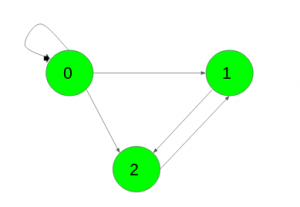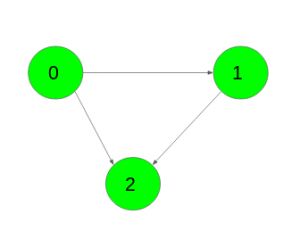# Find the number of paths of length K in a directed graph

• Difficulty Level : Medium
• Last Updated : 19 Jul, 2021

Given a directed, unweighted graph with N vertices and an integer K. The task is to find the number of paths of length K for each pair of vertices (u, v). Paths don’t have to be simple i.e. vertices and edges can be visited any number of times in a single path.
The graph is represented as adjacency matrix where the value G[i][j] = 1 indicates that there is an edge from vertex i to vertex j and G[i][j] = 0 indicates no edge from i to j.
Examples:

Input: K = 2,Output:
1 2 2
0 1 0
0 0 1
Number of paths from 0 to 0 of length k is 1({0->0->0})
Number of paths from 0 to 1 of length k are 2({0->0->1}, {0->2->1})
Number of paths from 0 to 2 of length k are 2({0->0->2}, {0->1->2})
Number of paths from 1 to 1 of length k is 1({1->2->1})
Number of paths from 2 to 2 of length k is 1({2->1->2})
Input: K = 3,Output:
1 0 0
0 1 0
0 0 1
Number of paths from 0 to 0 of length k is 1({0->1->2->0})
Number of paths from 1 to 1 of length k is 1({1->2->0->1})
Number of paths from 2 to 2 of length k is 1({2->1->0->2})

Prerequisite: Matrix exponentiation, Matrix multiplication
Approach: It is obvious that given adjacency matrix is the answer to the problem for the case k = 1. It contains the number of paths of length 1 between each pair of vertices.
Let’s assume that the answer for some k is Matk and the answer for k + 1 is Matk + 1
Matk + 1[i][j] = ∑p = 1NMatk[i][p]*G[p][j]
It is easy to see that the formula computes nothing other than the product of the matrices Matk and G i.e. Matk + 1 = Matk * G
Thus, the solution of the problem can be represented as Matk = G * G * … * G(k times) = Gk
Below is the implementation of the above approach:

## C++

 `// C++ implementation of the approach``#include ``using` `namespace` `std;` `#define N 3` `// Function to multiply two matrices``void` `multiply(``int` `a[][N], ``int` `b[][N], ``int` `res[][N])``{``    ``int` `mul[N][N];``    ``for` `(``int` `i = 0; i < N; i++) {``        ``for` `(``int` `j = 0; j < N; j++) {``            ``mul[i][j] = 0;``            ``for` `(``int` `k = 0; k < N; k++)``                ``mul[i][j] += a[i][k] * b[k][j];``        ``}``    ``}` `    ``// Storing the multiplication result in res[][]``    ``for` `(``int` `i = 0; i < N; i++)``        ``for` `(``int` `j = 0; j < N; j++)``            ``res[i][j] = mul[i][j];``}` `// Function to compute G raised to the power n``void` `power(``int` `G[N][N], ``int` `res[N][N], ``int` `n)``{` `    ``// Base condition``    ``if` `(n == 1) {``        ``for` `(``int` `i = 0; i < N; i++)``            ``for` `(``int` `j = 0; j < N; j++)``                ``res[i][j] = G[i][j];``        ``return``;``    ``}` `    ``// Recursion call for first half``    ``power(G, res, n / 2);` `    ``// Multiply two halves``    ``multiply(res, res, res);` `    ``// If n is odd``    ``if` `(n % 2 != 0)``        ``multiply(res, G, res);``}` `// Driver code``int` `main()``{``    ``int` `G[N][N] = { { 1, 1, 1 },``                    ``{ 0, 0, 1 },``                    ``{ 0, 1, 0 } };` `    ``int` `k = 2, res[N][N];` `    ``power(G, res, k);` `    ``for` `(``int` `i = 0; i < N; i++) {``        ``for` `(``int` `j = 0; j < N; j++)``            ``cout << res[i][j] << ``" "``;``        ``cout << ``"\n"``;``    ``}` `    ``return` `0;``}``// This Code is improved by cidacoder`

## Java

 `// Java implementation of the approach``class` `GFG``{``    ` `static` `int` `N = ``3``;` `// Function to multiply two matrices``static` `void` `multiply(``int` `a[][], ``int` `b[][], ``int` `res[][])``{``    ``int` `[][]mul = ``new` `int``[N][N];``    ``for` `(``int` `i = ``0``; i < N; i++)``    ``{``        ``for` `(``int` `j = ``0``; j < N; j++)``        ``{``            ``mul[i][j] = ``0``;``            ``for` `(``int` `k = ``0``; k < N; k++)``                ``mul[i][j] += a[i][k] * b[k][j];``        ``}``    ``}` `    ``// Storing the multiplication result in res[][]``    ``for` `(``int` `i = ``0``; i < N; i++)``        ``for` `(``int` `j = ``0``; j < N; j++)``            ``res[i][j] = mul[i][j];``}` `// Function to compute G raised to the power n``static` `void` `power(``int` `G[][], ``int` `res[][], ``int` `n)``{` `    ``// Base condition``    ``if` `(n == ``1``) {``        ``for` `(``int` `i = ``0``; i < N; i++)``            ``for` `(``int` `j = ``0``; j < N; j++)``                ``res[i][j] = G[i][j];``        ``return``;``    ``}` `    ``// Recursion call for first half``    ``power(G, res, n / ``2``);` `    ``multiply(res, res, res);` `    ``// If n is odd``    ``if` `(n % ``2` `!= ``0``)``        ``multiply(res, G, res);``}` `// Driver code``public` `static` `void` `main(String[] args)``{``    ``int` `G[][] = { { ``1``, ``1``, ``1` `},``                    ``{ ``0``, ``0``, ``1` `},``                    ``{ ``0``, ``1``, ``0` `} };` `    ``int` `k = ``2``;``    ``int` `[][]res = ``new` `int``[N][N];` `    ``power(G, res, k);` `    ``for` `(``int` `i = ``0``; i < N; i++)``    ``{``        ``for` `(``int` `j = ``0``; j < N; j++)``            ``System.out.print(res[i][j] + ``" "``);``        ``System.out.println(``""``);``    ``}``}``}` `// This code is contributed by 29AjayKumar``// This Code is improved by cidacoder`

## Python3

 `# Python3 implementation of the approach` `import` `numpy as np` `N ``=` `3` `# Function to multiply two matrices``def` `multiply(a, b, res) :` `    ``mul ``=` `np.zeros((N,N));``    ` `    ``for` `i ``in` `range``(N) :``        ``for` `j ``in` `range``(N) :``            ``mul[i][j] ``=` `0``;``            ``for` `k ``in` `range``(N) :``                ``mul[i][j] ``+``=` `a[i][k] ``*` `b[k][j];` `    ``# Storing the multiplication result in res[][]``    ``for` `i ``in` `range``(N) :``        ``for` `j ``in` `range``(N) :``            ``res[i][j] ``=` `mul[i][j];`  `# Function to compute G raised to the power n``def` `power(G, res, n) :``    ` `    ``# Base condition``    ``if` `(n ``=``=` `1``) :``        ``for` `i ``in` `range``(N) :``            ``for` `j ``in` `range``(N) :``                ``res[i][j] ``=` `G[i][j];``        ``return``;` `    ``# Recursion call for first half``    ``power(G, res, n ``/``/` `2``);` `    ``# Multiply two halves``    ``multiply(res, res, res);` `    ``# If n is odd``    ``if` `(n ``%` `2` `!``=` `0``) :``        ``multiply(res, G, res);` `# Driver code``if` `__name__ ``=``=` `"__main__"` `:` `    ``G ``=` `[``        ``[ ``1``, ``1``, ``1` `],``        ``[ ``0``, ``0``, ``1` `],``        ``[ ``0``, ``1``, ``0` `]``        ``];` `    ``k ``=` `2``;``    ``res ``=` `np.zeros((N,N));` `    ``power(G, res, k);` `    ``for` `i ``in` `range``(N) :``        ``for` `j ``in` `range``(N) :``            ``print``(res[i][j],end ``=` `" "``);``        ` `        ``print``()``        ` `# This code is contributed by AnkitRai01``# This Code is improved by cidacoder`

## C#

 `// C# implementation of the approach``using` `System;` `class` `GFG``{``    ` `static` `int` `N = 3;` `// Function to multiply two matrices``static` `void` `multiply(``int` `[,]a, ``int` `[,]b, ``int` `[,]res)``{``    ``int` `[,]mul = ``new` `int``[N,N];``    ``for` `(``int` `i = 0; i < N; i++)``    ``{``        ``for` `(``int` `j = 0; j < N; j++)``        ``{``            ``mul[i,j] = 0;``            ``for` `(``int` `k = 0; k < N; k++)``                ``mul[i,j] += a[i,k] * b[k,j];``        ``}``    ``}` `    ``// Storing the multiplication result in res[][]``    ``for` `(``int` `i = 0; i < N; i++)``        ``for` `(``int` `j = 0; j < N; j++)``            ``res[i,j] = mul[i,j];``}` `// Function to compute G raised to the power n``static` `void` `power(``int` `[,]G, ``int` `[,]res, ``int` `n)``{` `    ``// Base condition``    ``if` `(n == 1) {``        ``for` `(``int` `i = 0; i < N; i++)``            ``for` `(``int` `j = 0; j < N; j++)``                ``res[i,j] = G[i,j];``        ``return``;``    ``}` `    ``// Recursion call for first half``    ``power(G, res, n / 2);` `    ``// Multiply two halves``    ``multiply(res, res, res);` `    ``// If n is odd``    ``if` `(n % 2 != 0)``        ``multiply(res, G, res);``}` `// Driver code``public` `static` `void` `Main()``{``    ``int` `[,]G = { { 1, 1, 1 },``                    ``{ 0, 0, 1 },``                    ``{ 0, 1, 0 } };` `    ``int` `k = 2;``    ``int` `[,]res = ``new` `int``[N,N];` `    ``power(G, res, k);` `    ``for` `(``int` `i = 0; i < N; i++)``    ``{``        ``for` `(``int` `j = 0; j < N; j++)``            ``Console.Write(res[i,j] + ``" "``);``        ``Console.WriteLine(``""``);``    ``}``}``}` `// This code is contributed by anuj_67..``// This code is improved by cidacoder`

## Javascript

 ``

Output:

```1 2 2
0 1 0
0 0 1```

Time Complexity –
Since we have to multiply the adjacency matrix log(k) times (using matrix exponentiation), the time complexity of the algorithm is O((|V|^3)*log(k)), where V is the number of vertices, and k is the length of the path.

My Personal Notes arrow_drop_up